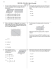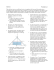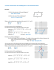# Algebra: Simplifying Algebraic Expressions, Expanding Brackets

## Transcription

Algebra: Simplifying Algebraic Expressions, Expanding Brackets
```Algebra: Simplifying Algebraic Expressions, Expanding Brackets,
Solving Linear Equations, Applications. KS3, KS4. Non-Calculator.
A. Simplify the following expressions:
1.
5.
8.
9.
10.
11.
12.
13.
14.
15.
16.
18.
20.
22.
24.
26.
28.
29.
30.
31.
mathsmalakiss.com
1
B. Expand the brackets and simplify where possible.
1. 4(x – 3)
2. 4(2x – 3)
3.
5.
7.
9.
11.
13.
15.
(x – 3)
16.
4(2x – 3)
17.
19.
21.
23.
25.
27.
28.
29.
31.
32.
33.
34.
35.
36.
37.
40.
mathsmalakiss.com
2
C. Solve the following equations:
1.
5.
7.
9.
10.
13.
16.
18.
20.
22.
24.
26.
28.
34.
35.
36.
38.
40.
mathsmalakiss.com
3
APPLICATIONS:
1. The width of a rectangle is x centimeters and its length is (x + 5) cm.
(x + 5)
Diagram not drawn to scale
x
(a) Write down an expression for the perimeter of the rectangle, giving your
The perimeter of the rectangle is 62 cm.
(b) Work out the length of the rectangle.
2. The diagram below is a rectangle. All measurements are in centimeters.
Diagram not drawn to scale
(2x + 10)
(x - 3 )
28 cm
(a) Work out the value of x.
(b) Hence, work out the perimeter and area of the rectangle.
mathsmalakiss.com
4
3. PQR is an isosceles triangle with PQ = PR, and angle QPR = x˚.
P
Angle PQR = (2x + 30)˚
Diagram not drawn to scale
x
PR = (2r + 3) cm
QR = (r + 3) cm
(2r + 3)
(2x + 30)˚
R
Q
(r + 3)
(a) Find an expression for the perimeter of the triangle in terms of r, giving
(b) Work out the value of r, if the perimeter is 49 cm.
(c) Work out the value of x.
4. The area of the right-angled triangle is equal to the area of the rectangle.
Work out the value of x. All measurements are in centimeters.
Diagrams not drawn to scale
10
x
25
15
20
mathsmalakiss.com
5
mathsmalakiss.com
6
mathsmalakiss.com
7
mathsmalakiss.com
8
mathsmalakiss.com
9
mathsmalakiss.com
10
The Length =
mathsmalakiss.com
11
Hence the width =
Area =
I hope you find this useful. If you find any errors, please let me know.
mathsmalakiss.com
12
```

### Ch. 7 Test Study Guide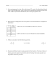### In the punalu`u lo`i, there is a taro patch that is a Rectangle. We need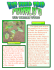### Open-ended problems with purpose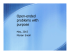### Geo BB1 & 3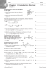### 10th Wksht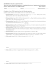### Algebra with shapes How to . . .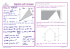### Pencil Box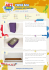### Math 220 Sample Exam 4### Hipwee Social Marketing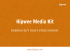### Perimeter and Area Now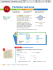### MATH1013 Calculus I, 2013-14 Spring Tutorial Worksheet 10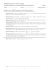### Lesson 23 Homework 3 7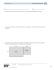### Integrated Math II Honors w/ solutions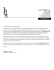### Perimeter and Area Now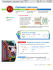### Shadows in FlexiSIGN Pro ver 7.6 - Digital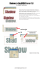### INTERNATIONAL INDI Mathematics worksheet Name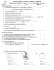### Perimeter Security Market in North America 2015-2019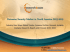### Document 6532627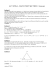### Reteach Workbook Chapter 11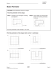### File - Waller Junior High Math